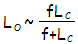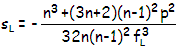telescopeѲptics.net          ▪▪▪▪                                                                               CONTENTS

# 4.8.6. DALL AND ROSS NULL TESTS

Dall null test uses single lens placed between light source and mirror on test, with the mirror tilted as needed to clear the lens from the path of the converging beam (FIG. 57). The lens induces nearly identical amount of spherical aberration of opposite sign to that of a mirror of specified conic, making possible to quickly assess the accuracy of mirror surface by observing how well it "nulls"; that is, whether or not the resulting focus is tight enough to produce darkening evenly spread across the surface when the light is intercepted at the focus point (the lens can also be placed in the converging cone, with very similar results; the advantage of such a setup is that it ensures that the entire mirror surface is tested).FIGURE 57: Usually done in combination with Foucault test apparatus, Dall null test has the lens tailored to fit a mirror imaging point at the center of curvature. Thus the lens and light source are positioned so that the refracted rays projected backwards focus at the mirror's center of curvature. In other words. the null lens produces diverging cone nearly coinciding with that of the mirror focusing at the center of curvature, thus producing near-identical aberration at the mirror. On its part, the lens induces nearly identical aberration of opposite sign, which results in a formation of near-perfect focus that will make possible to null accurately made surface.

For given lens separation from the mirror center of curvature LC, this setup requires lens-to-source separationwith ƒ being the lens focal length, given by ƒ=R/(n-1) for plano-convex, and ƒ=R/2(n-1) for equi-convex lens, where R is the surface radius of curvature, and n the index of refraction. This initial location not necessarily results in the aberration offset, and usually requires minor adjustment (the figure is usually more accurate when measured from the center of the lens, due to lens thickness being a factor not accounted for in the Gaussian lens formula that the relation is obtained from). Since lens' spherical aberration changes with R3, relatively small variations lead to the desired aberration level, with the change in effective lens diameter due to needed axial adjustments having relatively small effect on the aberration.

Main limitation of the Dall null test results from the need to bypass correcting lens with the converging cone. It requires tilting the mirror (it can also be thought of as placing light source off the mirror optical axis), which inevitably induces astigmatism. For mirror with the stop at surface, it is independent of object distance, thus as given by Eq. 72. In terms of the maximum allowable RMS error of astigmatism ω, the corresponding tilt angle τ is given by:

τ = (Fω/D)1/2/6.8

in radians. Taking ω=0.03 waves RMS of astigmatism as the maximum acceptable level (comparable to 1/10 wave P-V of spherical aberration), gives the maximum acceptable tilt angle τ=F/D/39, with F being the mirror infinity F-number, and D the aperture diameter in mm. It, in turn, determines the width of converging cone at the point where it splits off the diverging cone, at R/(4Fτ+1) from the mirror, as Ø=FτD/(Fτ+0.25). This is the approximate upper limit for the lens diameter, with the actual lens being somewhat closer to the focus, to prevent the converging cone from passing close to its edges (it is recommendable to use less than 2/3 of lens radius, to avoid its often less accurate outer zone).

For an average 300mm ƒ/5 mirror, maximum acceptable tilt angle is τ=0.0033 (0.19°), with near-maximum lens diameter Ømax~20mm. The point of cone separation is, therefore, about 190mm from mirror's center of curvature, but the actual lens shouldn't be farther than 140mm from it. Needed lens-to-source separation LO is dependent on the lens focal length ƒL and lens-to-c.o.c. separation LC (which is the image distance for the lens, and object distance for the mirror). As given above, LO=LCƒL/(LC+ƒL).

The ƒL value is chosen so that the peak aberration coefficient for Seidel spherical aberration sLdL4 of the lens is nearly equal to the mirror peak aberration coefficient, SM=KDM4/64RM3 (peak aberration coefficient for spherical aberration equals the P-V wavefront error at paraxial focus), and of opposite sign. Taking equi-convex lens with shape factor q=0, to simplify Eq. 8, the lens aberration coefficient becomes:Substituting for n~1.52 for BK7 glass further simplifies the relation to sL=(3.5+1.8p2)/13.2ƒL3. Taking L=-140mm as the farthest lens-to-center-of-curvature separation that allows to leave out the outer lens area (distance from lens to the center of curvature is numerically negative since to the left of the surface), position factor p becomes p=1+ƒL/70.

Since there is no simple way to extract ƒL at this point, the simplest way to get a sense of direction is to guess for ƒL and see the result. Taking ƒL=70mm and substituting for p=2 gives sL=1/1.23fL3=1/420,000. With the effective aperture radius dL=7mm, the lens peak aberration coefficient is SL=-74/420,000=-0.0057. Comparison with the mirror peak aberration coefficient SM=0.0047, shows that lens focal length needs to be relaxed by a (57/47) factor, resulting in ƒL~75mm. This approximates needed lens-to-source separation as LO~49mm.

From this point on, it is best to refine a setup with ray trace. Final optimization for this particular setup in OSLO, including minimizing spherical aberration by balancing its higher- and lower-order components, resulted in the lens focal length ƒL=77mm (80mm lens surface radii), and lens-to-source separation LO=47.6mm. The RMS wavefront error of this near-perfect setup is 0.038 (546nm unit), with the excess over 0.03 wave RMS of mirror tilt caused astigmatism coming from the minimized higher-order spherical aberration. It is barely passable, not only because it exceeds 0.03 wave RMS limit (0.038 wave RMS is comparable to 1/8 wave P-V of lower-order spherical), but also because it uses about 70% of the lens radius. More so considering that the error in an actual setup is all but certain to be larger, and that it will also increase in proportion with the aperture.

The most sensitive to axial displacement is lens-to-source separation; in this particular setup, 1mm spacing error generated about 0.08 wave RMS of additional aberration, setting the tolerance at ±0.2mm at most. Error in the lens-to-mirror spacing is much more forgiving, with 10mm larger separation raising the error to 0.04 wave RMS, and as much of reduced separation only to 0.036 wave.The above example suggests that the Dall null test is probably not recommendable for larger and/or faster mirrors than ~300mm ƒ/5. This limits its usefulness, since it is with those larger and faster mirrors that null test comes particularly handy. For smaller and slower mirrors, the astigmatism and the overall error of the Dall null test can be kept negligibly low. For larger mirrors and relative apertures, Ross null test is a better option. It also uses positive lens to cancel spherical aberration of a mirror, but both, lens and source are centered on mirror's axis, with the light passing through the lens twice before coming to a focus (obviously, similarly to double pass test, some type of beam splitter is necessary to separate the focus from the source).

Same basic relations for lens and source locations used for Dall null test can be used for the Ross; the main difference is that the lens aberration coefficient in the Ross null is a sum of the coefficients for each light pass. Fortunately, there is no need to fiddle with numbers. An excellent Ross null test shareware program written by Doug George, and rewritten in BASIC by James Lerch, gives all the information needed to conduct the test.

4.8.5. Hindle sphere test   ▐    4.8.7. Offner null test